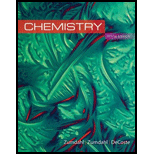# At 25°c, K = 0.090 for the reaction H 2 O ( g ) + Cl 2 O ( g ) ⇌ 2 HOCI ( g ) Calculate the concentrations of all species at equilibrium for each of the following cases. a . 1.0 g H 2 O and 2.0 g Cl 2 O are mixed in a 1.0-L flask. b . 1.0 mole of pure HOCI is placed in a 2.0-L flask.### Chemistry

10th Edition
Steven S. Zumdahl + 2 others
Publisher: Cengage Learning
ISBN: 9781305957404

#### Solutions

Chapter
Section### Chemistry

10th Edition
Steven S. Zumdahl + 2 others
Publisher: Cengage Learning
ISBN: 9781305957404
Chapter 13, Problem 60E
Textbook Problem
900 views

## At 25°c, K = 0.090 for the reaction H 2 O ( g ) + Cl 2 O ( g ) ⇌ 2 HOCI ( g ) Calculate the concentrations of all species at equilibrium for each of the following cases.a. 1.0 g H2O and 2.0 g Cl2O are mixed in a 1.0-L flask.b. 1.0 mole of pure HOCI is placed in a 2.0-L flask.

(a)

Interpretation Introduction

Interpretation: The value of the equilibrium constant for the reaction between H2O and Cl2O and the initial mass of the species involved is given. The equilibrium concentrations of the three gases involved in the system are to be calculated.

Concept introduction: The state when the reactants involved in a chemical reaction and the products formed in the reaction exist in concentrations having no further tendency to change is known as an equilibrium state of the reaction. When the equilibrium constant is expressed in terms of concentration, it is represented as K .

To determine: The equilibrium concentrations of the three gases involved in the system

### Explanation of Solution

Given

The stated reaction is,

H2O(g)+Cl2O(g)2HOCl(g)

The initial mass of H2O(g) is 1.0g .

The initial mass of Cl2O(g) is 2.0g .

The equilibrium constant (K) value is 0.090 .

The volume of the container is 1.00L .

The molar mass of H2O=2H+O=2(1)+16=18g/mol

The molar mass of Cl2O=2Cl+O=2(35.5)+16=87g/mol

Formula

The number of moles of a substance is calculated by the formula,

Numberofmoles=GivenmassMolarmass

Substitute the values of the given mass and molar mass of H2O and Cl2O in the above expression.

For H2O ,

NumberofmolesofH2O=1.0g18.0g/mol=0.056mol

For Cl2O ,

NumberofmolesofCl2O=2.0g87.0g/mol=0.023mol

The concentration of a reactant is calculated by the formula,

Concentration=MolesVolume(L)

The initial concentration of H2O is calculated by the formula,

Substitute the given values of the number of moles of H2O and the volume of the flask in the above expression.

ConcentrationofH2O=0.056moles1.00L=0.056M

The initial concentration of Cl2O is calculated by the formula,

Substitute the given values of the number of moles of Cl2O and the volume of the flask in the above expression.

ConcentrationofCl2O=0.023moles1.00L=0.023M

To determine: The equilibrium concentrations of the three gases involved in the system.

Explanation:

The concentration of H2O and Cl2O consumed is assumed to be x .

The equilibrium concentrations are represented as,

H2O(g)+Cl2O(g)2HOCl(g)Initialconcentration0.0560.0230Change-x-x+2xEquilibriumconcentration0.056-x0.023-x2x

At equilibrium, the equilibrium ratio is expressed by the formula,

K=ConcentrationofproductsConcentrationofreactants

Where,

• K is the equilibrium constant.

The equilibrium ratio for the given reaction is,

K=[HOCl]2[H2O][Cl2O] (1)

According to the formulated ICE table,

The equilibrium concentration of H2O(g) is (0

(b)

Interpretation Introduction

Interpretation: The value of the equilibrium constant for the reaction between H2O and Cl2O and the initial mass of the species involved is given. The equilibrium concentrations of the three gases involved in the system are to be calculated.

Concept introduction: The state when the reactants involved in a chemical reaction and the products formed in the reaction exist in concentrations having no further tendency to change is known as an equilibrium state of the reaction. When the equilibrium constant is expressed in terms of concentration, it is represented as K .

To determine: The equilibrium concentrations of the three gases involved in the system.

### Still sussing out bartleby?

Check out a sample textbook solution.

See a sample solution

#### The Solution to Your Study Problems

Bartleby provides explanations to thousands of textbook problems written by our experts, many with advanced degrees!

Get Started

Find more solutions based on key concepts
Define the terms matter and mass. What is the difference between mass and weight?

General Chemistry - Standalone book (MindTap Course List)

The Academy of Nutrition and Dietetics recommends fluoride-free water for the U.S. population. T F

Nutrition: Concepts and Controversies - Standalone book (MindTap Course List)

What is the difference between a hypothesis , theory, and a law?

Horizons: Exploring the Universe (MindTap Course List)

What is oxyhemoglobin? Where does it form?

Human Biology (MindTap Course List)

U.S. life expectancy at birth is the highest in the world. _____ True _____ False

Nutrition Through the Life Cycle (MindTap Course List)

A sphere of radius 2a is made of a nonconducting material that has a uniform volume charge density . Assume the...

Physics for Scientists and Engineers, Technology Update (No access codes included)

The __________ circuit carries blood to and from lungs. a. systemic b. pulmonary

Biology: The Unity and Diversity of Life (MindTap Course List)

What purpose do the colors in a false-color image or map serve?

Foundations of Astronomy (MindTap Course List)

What was the key insight that Hess and Wilson brought to the discussion?

Oceanography: An Invitation To Marine Science, Loose-leaf Versin